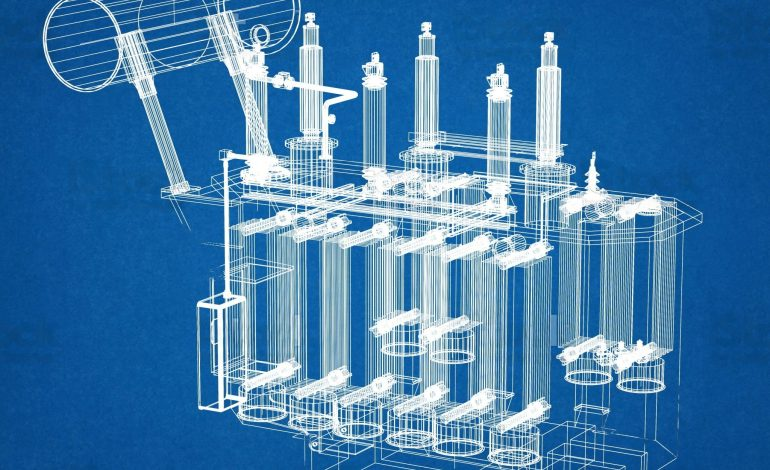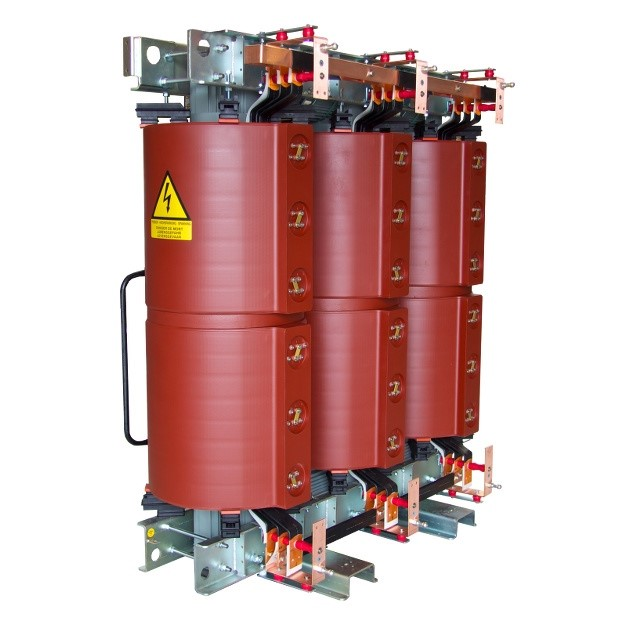Contact Number +90 536 226 00 45

## Dry Type Transformer Percent Impedance DefinitionIntroduction

The percent impedance is the percent voltage required to circulate rated current flow through one transformer winding when another winding is short-circuited at the rated voltage tap at rated frequency. %Z is related to the short circuit capacity of the transformer during short circuit conditions.Fig. 1. Dry Type Transformer

For a two-winding transformer with a 5% impedance, it would require 5% input voltage applied on the high voltage winding to draw 100% rated current on the secondary winding when the secondary winding is short-circuited. If 100% rated voltage is applied to the high voltage winding, approximately 20X rated current would flow in the secondary winding when the secondary winding is short-circuited.

Table 1. Impedance Levels

 Based kVA Minimum Impedance, % 0-150 Manufacturer’s Standard 151-300 4 301-600 5 601-2500 6 2501-5000 6.5 5001-7500 7.5 7501-10000 8.5 Above 10000 9.5

Important Notes

1. The impedance of a two-winding transformer shall not vary from the guaranteed value by more that ± 7.5%
2. The impedance of a transformer having three or more windings or having zig-zag windings shall not vary from the guaranteed value by more than ± 10%
3. The impedance of an autotransformer shall not vary from the guaranteed value by more than ± 10%
4. The difference of impedances between transformers of the same design shall not exceed 10% of the guaranteed values
5. Differences of impedance between autotransformers of the same design shall not exceed 10% of the guaranteed values

Impedance vs. Percent Impedance

Impedance is defined, in the Standard Handbook for Electrical Engineers, as “the apparent resistance of an alternating current circuit or path… the vector sum of the resistance and reactance of the path”. Impedance may be comprised of resistance, capacitive reactance, and inductive reactance, and is expressed in ohms.

From the perspective of a load, the total input impedance may include the impedance of the upstream generator, transformer, line reactor, and conductors.

Sample calculations for a three-phase transformer rated 500kVA, 4160:480, 60Hz, 6% impedance:

Transformer reactance  Xt= (kV2 /MVA) x %Z/100 = (0.48  / 0.5) x 0.06 = 0.027648 ohms

Approximate available short circuit current = 480/(1.732 x 0.027648) = 10,023.7 amps

Effective Percent Impedance

Effective impedance is the relative impedance of a reactor or transformer under actual operating conditions. Since smaller (kVA) loads have higher impedance and thus draw lower current than larger (kVA) loads, the internal ohms of a reactor or transformer represent a smaller percentage of the load impedance for a small (kVA) load than for a large load.

The value in ohms will cause a lower voltage drop when less than rated reactor or transformer current is flowing. If the load is only one half the rated current, then the voltage drop across the impedance will be one half of the rated voltage drop.

Sample calculations for a three-phase transformer rated 500kVA, 4160:480, 60Hz, 6% impedance:

Xt = (kV2 /MVA) x %Z/100 = (0.482 / 0.5) x 0.06 = 0.027648 ohms

Rated secondary current = 500,000 / (480 x 1.732) = 601.4 amps

Actual Load current = 300 amps

Voltage drop at actual load = 300 x 1.732 x 0.027648 = 14.36 volts (14.36 / 480 = 0.0299, or 3% of 480 volts)

Effective percent impedance = 6% x (300 / 601.4) = 2.99%

Conclusion

Percent impedance is an important parameter that determines a transformer’s short-circuit capacity and the voltage drop during high current flow. The percent impedance value is a crucial factor to consider during transformer design and operation. Additionally, it is necessary for ensuring the safety of electrical systems by using it in short-circuit calculations. Therefore, the significance of percent impedance holds great importance in the field of electrical engineering.